【RAY TRACING THE REST OF YOUR LIFE 超详解】 光线追踪 3-6 直接光源采样

Chapter7 Sample Lights Directly

Preface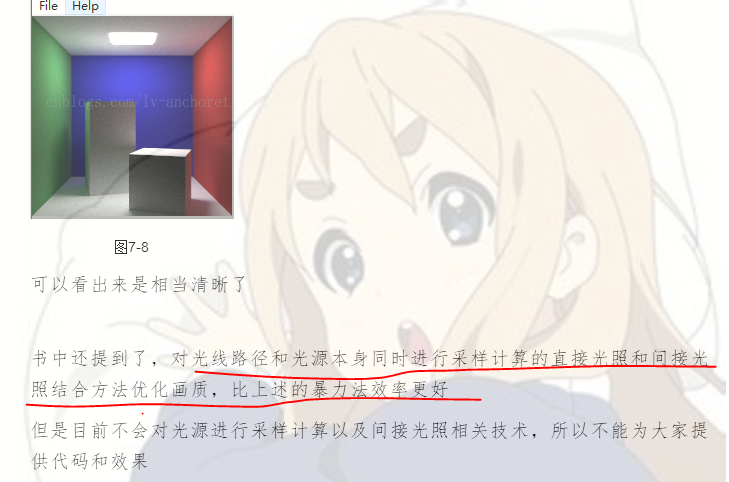1.微分

2.立体角 （蒙特卡罗（三））

content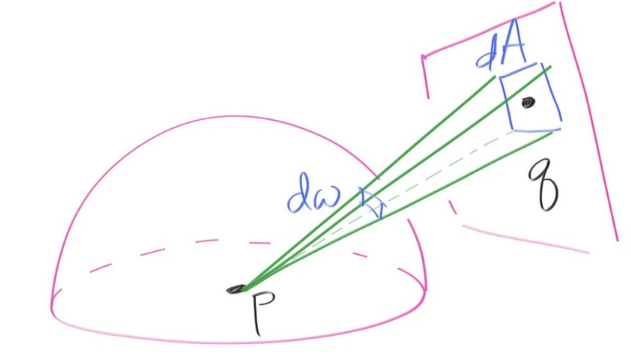？为什么老是提到单位球体呢？？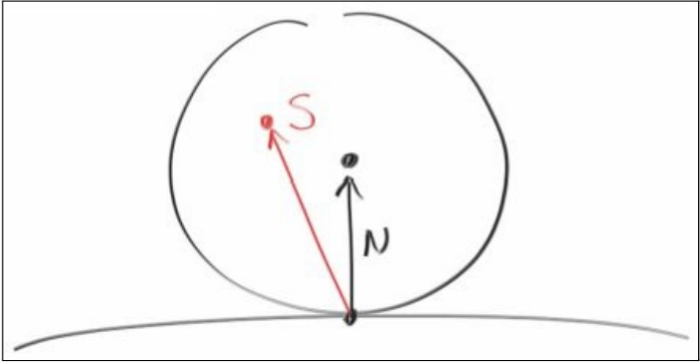p_q(q)*dA（采样比例乘以微分区域）,也就是dA/A

p(direction)*dΩ

= dA cosα / （distance（p,q^2）

p(direction) * cosα * dA / (distance(p,q)^2) = p_q(q) * dA = dA / A

p(direction) = distance(p,q)^2 / (cosα * A)

list[cnt++] = new xz_rect(200, 350, 220, 340, 550, light);

rtvec lerp(const ray& sight, intersect* world, int depth)
{
hitInfo info;
if (world->hit(sight, (rtvar)0.001, rtInf(), info))
{
ray scattered;
rtvec emitted = info._materialp->emitted(info._u, info._v, info._p);
rtvar pdf;
rtvec albedo;
if (depth < 50 && info._materialp->scatter(sight, info, albedo, scattered, pdf))
{
rtvec on_light = rtvec(213 + lvgm::rand01() * (343 - 213), 554, 227 + lvgm::rand01() * (332 - 227));
rtvec to_light = on_light - info._p;
double distance_squared = to_light.squar();
to_light.self_unitization();
if (dot(to_light, info._n) < 0)
return emitted;
double light_area = (343 - 213)*(332 - 227);
double light_cosine = fabs(to_light.y());
if (light_cosine < 1e-6)
return emitted;
pdf = distance_squared / (light_cosine*light_area);
scattered = ray(info._p, to_light, sight.time());
return emitted + albedo *info._materialp->scatter_pdf(sight, info, scattered)*lerp(scattered, world, depth + 1) / pdf;
}
else
return emitted;
}
else
return rtvec();
}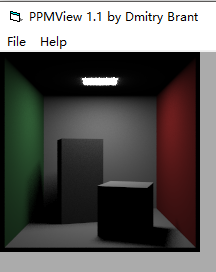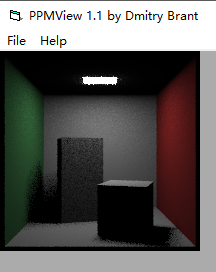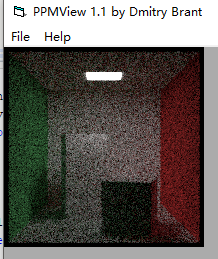virtual rtvec emitted(const ray& rIn, const hitInfo& info, const rtvar u, rtvar v, const rtvec& p)const
{
if(dot(info._n,rIn.direction())<0.)
return _emit->value(u, v, p);
else
return rtvec();
}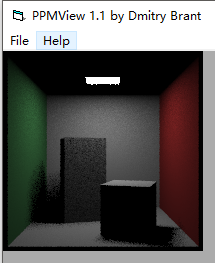posted @ 2019-03-14 23:58 林-兮 阅读(...) 评论(...) 编辑 收藏